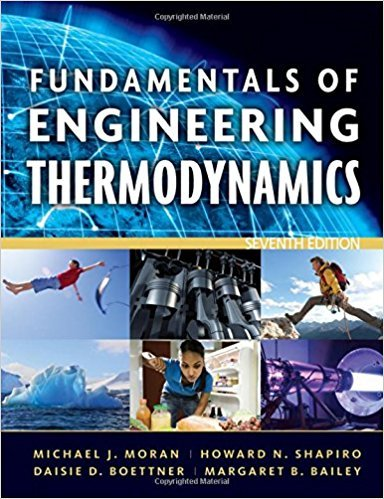×
Get Full Access to Fundamentals Of Engineering Thermodynamics - 7 Edition - Chapter 2 - Problem 26p
Get Full Access to Fundamentals Of Engineering Thermodynamics - 7 Edition - Chapter 2 - Problem 26p

×

# Evaluating WorkCarbon dioxide (CO2) gas within aISBN: 9780470495902 50

## Solution for problem 26P Chapter 2

Fundamentals of Engineering Thermodynamics | 7th Edition

• Textbook Solutions
• 2901 Step-by-step solutions solved by professors and subject experts
• Get 24/7 help from StudySoup virtual teaching assistantsFundamentals of Engineering Thermodynamics | 7th Edition

4 5 1 418 Reviews
10
2
Problem 26P

Problem 26P

Evaluating Work

Carbon dioxide (CO2) gas within a piston-cylinder assembly undergoes an expansion from a state where p1 = 20 lbf/in.2, V1 = 0.5 ft3 to a state where p2 = 5 lbf/in.2, V2 = 2.5 ft3. The relationship between pressure and volume during the process is p = A + BV, where A and B are constants.

(a) For the CO2, evaluate the work, in ft · lbf and Btu.

(b) Evaluate A, in lbf/in.2, and B, in (lbf/in.2)/ft3.

Step-by-Step Solution:

Step 1 of 3

Part a

We are required to calculate the work done for the Carbon Dioxide gas.

Given data:20 lbf/in20.5 ft35 lbf/in22.5 ft3

Step 2 of 2

##### ISBN: 9780470495902

Fundamentals of Engineering Thermodynamics was written by and is associated to the ISBN: 9780470495902. This textbook survival guide was created for the textbook: Fundamentals of Engineering Thermodynamics, edition: 7. The full step-by-step solution to problem: 26P from chapter: 2 was answered by , our top Engineering and Tech solution expert on 07/20/17, 09:01AM. Since the solution to 26P from 2 chapter was answered, more than 343 students have viewed the full step-by-step answer. This full solution covers the following key subjects: lbf, Where, state, evaluate, work. This expansive textbook survival guide covers 14 chapters, and 1501 solutions. The answer to “Evaluating WorkCarbon dioxide (CO2) gas within a piston-cylinder assembly undergoes an expansion from a state where p1 = 20 lbf/in.2, V1 = 0.5 ft3 to a state where p2 = 5 lbf/in.2, V2 = 2.5 ft3. The relationship between pressure and volume during the process is p = A + BV, where A and B are constants.(a) For the CO2, evaluate the work, in ft · lbf and Btu.________________(b) Evaluate A, in lbf/in.2, and B, in (lbf/in.2)/ft3.” is broken down into a number of easy to follow steps, and 77 words.

Unlock Textbook Solution